# Tangent Galvanometer

\$200.00

Code Number: Code: P0210211

Brand: AELAB

#### Purpose:

• To investigate the magnetic field at the center of a current-carrying loop of wire.
• To verify the relationship between the current, I, the number of turns, N, and the magnetic field, B, inside a current loop.
• To determine the strength of Horizontal Part of Earth's magnetic field.
- +

# All will be printed in English Language

## Tangent Galvanometer include:

- Wire frame: Diameter 180mm x height 26mm

- Base Dimension: 215 x 156 x 26mm

- Number of wire turn: 100, 200 and 300 turn, copper wire dia. 0.5mm. Number of turn is selected by rotary switch

- Large compass: Diameter 100mm, at the center of wire frame.

- DC Current throught wire loops can be adjustable. Max. current is 200mA to protect wire loops.

- Input source: 6 - 12VDC

- Multimeter

## Principles of Tangent Galvanometer:

The earth is surrounded by a magnetic field, the earth is like a giant magnet. The magnetic field lines go out from the north pole and into the south pole. At a point near the ground, the B-field is considered as a combination of two components: the horizontal magnetic field and the vertical magnetic field (below figure).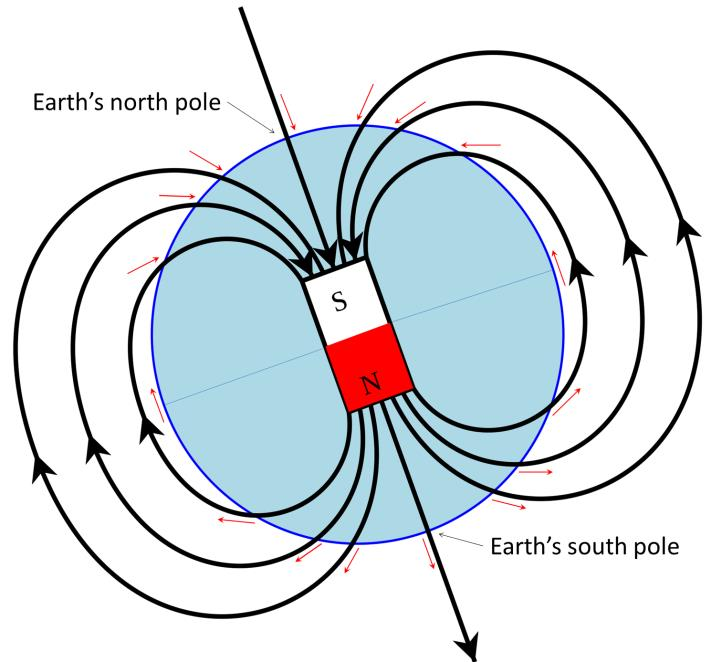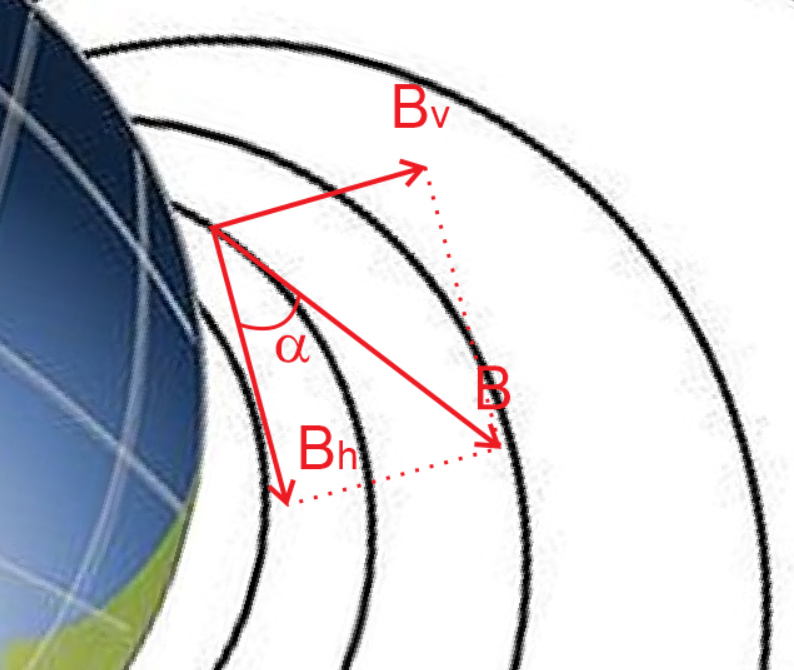Angle is the angle of inclination of the magnetic field relative to the horizontal.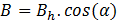The Tangent Galvanometer is used to determine the direction and magnitude of the Earth's magnetic field horizontally at a position. The horizontal component is determined, measuring the rotation angle of the compass needle when we apply an external magnetic field to the coil, thereby calculating the magnitude of the magnetic field.

When a current is applied to the coil, the coil of galvanometer generates a magnetic field Bloop.

The magnetic needle will be affected by the earth magnetic field in the Bh and the magnetic field caused by the wire loop Bloop. Needle will rotate angle β.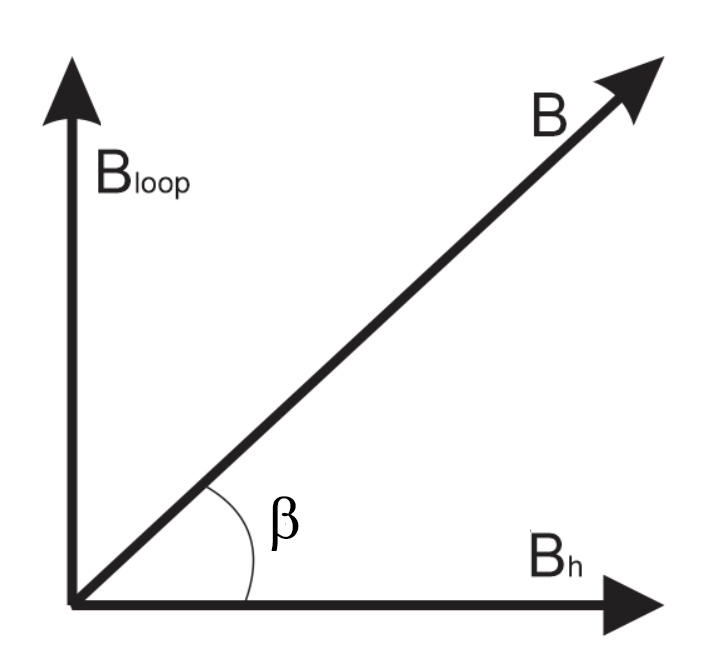Then: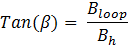(1)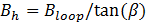(2)

We have formula  Bloop below: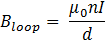(3)

In which: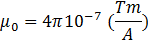(4)

d: Diameter loops

n: Number of loops

I: Current through loops

Then: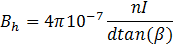(5)

## Procedure:

Step 1: Turn the galvanometer so that the needle of the compass is in the wireframe plane. That means the needle and the wireframe is in a plane (Picture below)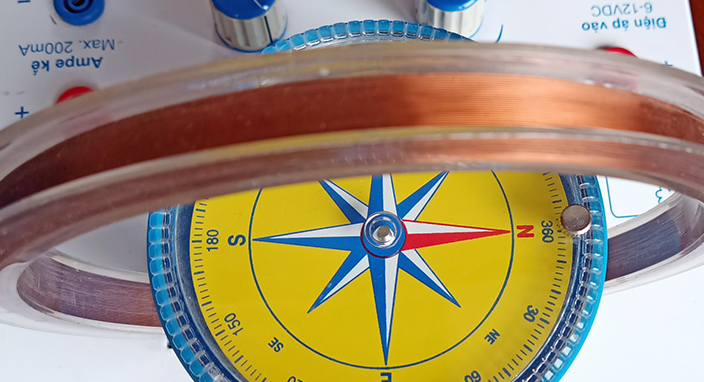.

Tangent Galvanometer: Compass

Question: Why do you have to arrange the compass needle in the wire frame plane ???

Answer: Then the magnetic field generated by the coil Bloop will be perpendicular to the earth magnetic field Bh.

Question: Why do you have to rotate the compass needle in the wire frame plane ???

Answer: Then the magnetic field generated by the Bloop coil will be perpendicular to the earth magnetic field Bh.

And then, we can use formula (1):Step 2: Adjust the needle do not touch the glass of the compass (balanced, can rotate freely without getting stuck).

Step 3: Plug the cords as shown in the picture: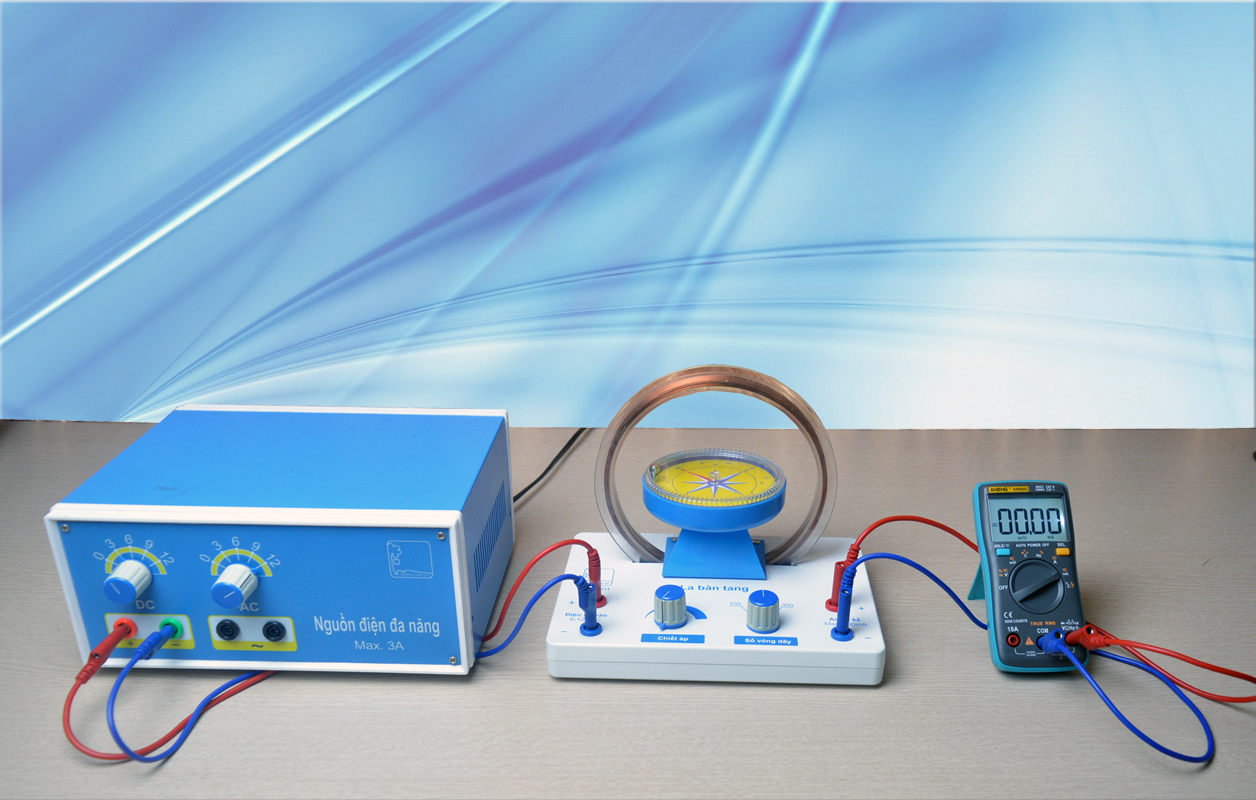Tangent Galvanometer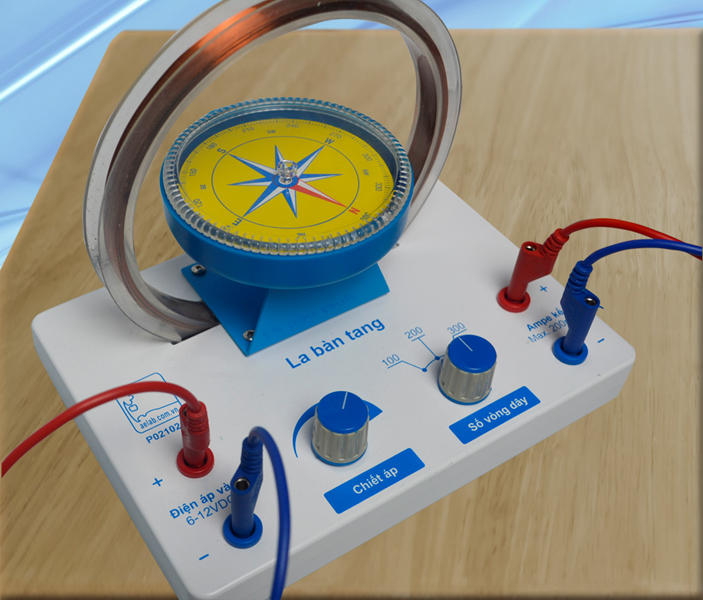Tangent Galvanometer

Step 4: Turn the potentiometer to the left (the current in the coil is 0). switch the knob to 300 turns. Power supply from 6V or 12V DC (Recommended to 6V)

Step 5: Turn on the power.

- Slowly increase the potentiometer so that the compass needle deviates β = 45 degrees. Record current value. we reduce the potentiometer to 0 again

- Switch the current to the potentiometer. We increase the current again so that the needle deviates 45 degrees (in the opposite direction). Record current value.

- We repeat the experiment with 100 turns, 200 turns.

Question: why do you do it with 45 degree deviation? Is it possible to have a 30 degree angle or another angle ???

Answer: This is a difficult question to explain to high school students but also easy to explain to college students.

Compass sensitivity: This is the ability of the compass needle to rotate an angle β per unit of current flowing.

Sensitivity = dβ / di.

After a while proved by a series of complex equations, the result is: Sens sensitivity is greatest when β = 45 degrees.

Step 6: Data processing

- Calculate the average value of the current ITB

- Based on formula (5) we calculate the composition of the horizontal earth magnetic field Bh: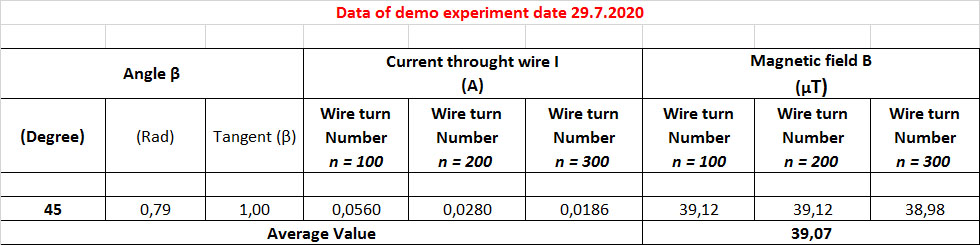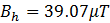According to value reported, Horizontal magnetic field in range:  25 µT - 75 µTTangent Galvanometer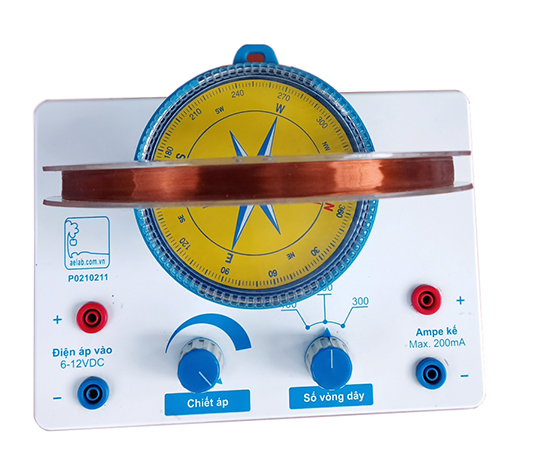Tangent Galvanometer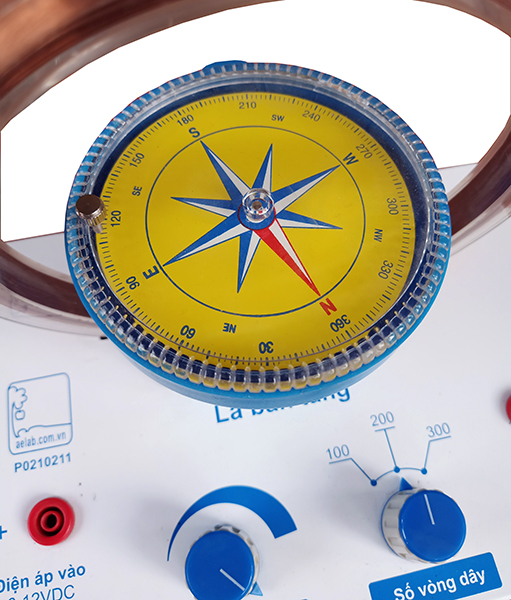Tangent Galvanometer: CompassTangent Galvanometer: English index